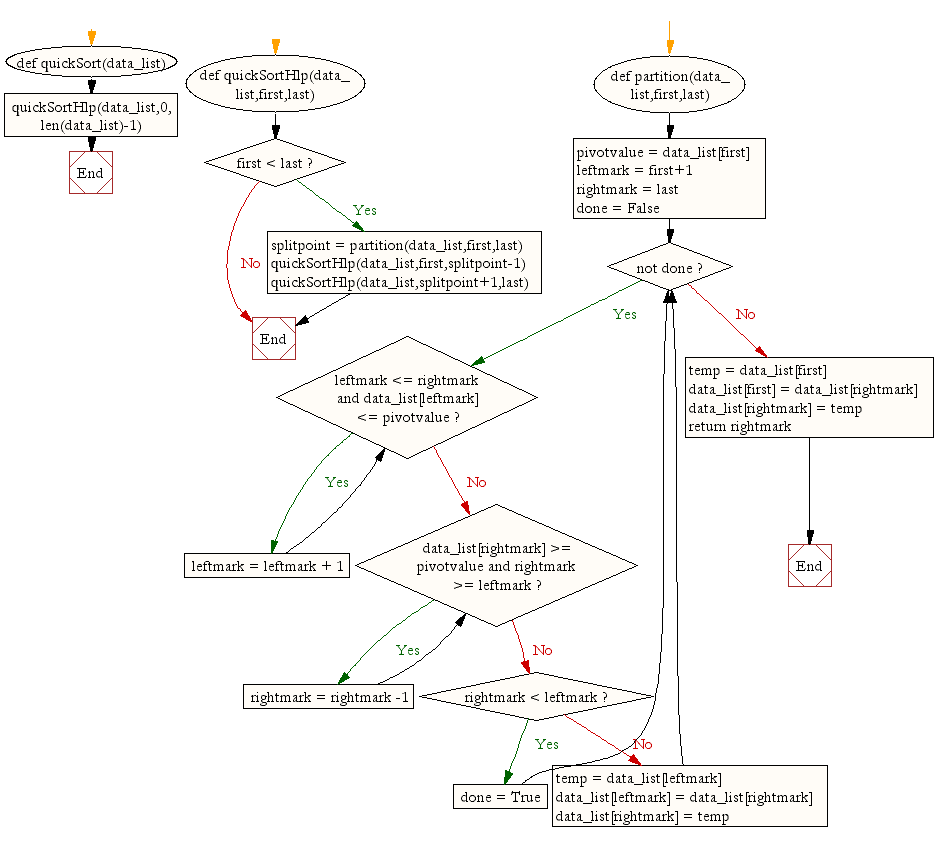﻿ Python: Quick sort - w3resource# Python: Quick sort

## Python Search and Sorting: Exercise-9 with Solution

Write a Python program to sort a list of elements using the quick sort algorithm.
Note : According to Wikipedia "Quicksort is a comparison sort, meaning that it can sort items of any type for which a "less-than" relation (formally, a total order) is defined. In efficient implementations it is not a stable sort, meaning that the relative order of equal sort items is not preserved. Quicksort can operate in-place on an array, requiring small additional amounts of memory to perform the sorting."

Sample Solution:

Python Code:

``````def quickSort(data_list):
quickSortHlp(data_list,0,len(data_list)-1)

def quickSortHlp(data_list,first,last):
if first < last:

splitpoint = partition(data_list,first,last)

quickSortHlp(data_list,first,splitpoint-1)
quickSortHlp(data_list,splitpoint+1,last)

def partition(data_list,first,last):
pivotvalue = data_list[first]

leftmark = first+1
rightmark = last

done = False
while not done:

while leftmark <= rightmark and data_list[leftmark] <= pivotvalue:
leftmark = leftmark + 1

while data_list[rightmark] >= pivotvalue and rightmark >= leftmark:
rightmark = rightmark -1

if rightmark < leftmark:
done = True
else:
temp = data_list[leftmark]
data_list[leftmark] = data_list[rightmark]
data_list[rightmark] = temp

temp = data_list[first]
data_list[first] = data_list[rightmark]
data_list[rightmark] = temp

return rightmark

data_list = [54,26,93,17,77,31,44,55,20]
quickSort(data_list)
print(data_list)
```
```

Sample Output:

```[17, 20, 26, 31, 44, 54, 55, 77, 93]
```

Flowchart:## Visualize Python code execution:

The following tool visualize what the computer is doing step-by-step as it executes the said program:

Python Code Editor :

What is the difficulty level of this exercise?

Test your Programming skills with w3resource's quiz.

﻿

## Python: Tips of the Day

Use Reversed() In for Loops:

```>>> tasks = ['laundry', 'picking up kids', 'gardening', 'cooking']